## research papersBIOLOGICALCRYSTALLOGRAPHY
ISSN: 1399-0047
Volume 57| Part 10| October 2001| Pages 1355-1359

## Rotations and rotation matrices

aMRC Laboratory of Molecular Biology, Hills Road, Cambridge CB2 2QH, England
*Correspondence e-mail: pre@mrc-lmb.cam.ac.uk

(Received 13 February 2001; accepted 12 June 2001)

In molecular replacement, a model, described as a list of orthogonal coordinates, is to be moved into a new position and orientation. The orthogonal coordinate system also has to be related to the crystallographic system, which may not be orthogonal, and crystallographic symmetry must be considered, which applies to coordinates expressed as fractions of the unit cell. Elementary properties of rotation matrices and their representation as polar or Eulerian angles are discussed.

### 1. Introduction

In the search process implicit in molecular replacement, a known model structure is rotated and translated into all possible orientations and positions for comparison with diffraction data for an unknown structure. This needs a mathematical description of the rotation and translation operations. In a Cartesian coordinate system, translations are straightforward, but rotations are not and their description is often confusing for the novice. This paper tries to provide an elementary introduction to rotations. This material is discussed with much more rigour and much greater detail in many places, including mathematical textbooks and in several articles in International Tables for Crystallography, Volume B, notably in the article by Diamond (1993).

### 2. Rotations and translations

The transformation of a positional vector xi describing the ith atom in a molecule can be written as a multiplication of the vector by a rotation matrix R and addition of a translation vector t. For this purpose, we work in an orthogonal Cartesian system in ångströms: conversion to fractional crystallographic coordinates is discussed in §6. The new positional vector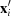is then given by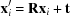for each atom i = 1, n, where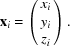Translations are not usually confusing, except that the order of rotation and translation matters. Rotating then translating by a vector t is not the same as translating by a vector s then rotating: the rotation operation remains the same but the translation vector needs to be rotated,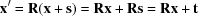i.e.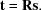### 3. Rotation matrices

A rotation matrix transforms the set of coordinates representing a three-dimensional object, in an orthogonal Cartesian frame, without changing its shape or size, i.e. the length of any vector and the angle between any pair of vectors are unchanged. Such a matrix is called orthonormal and has several properties which follow from this definition.

#### 3.1. The columns of a rotation matrix are orthogonal unit vectors

A rotation matrix may transform any set of vectors, so we can consider transforming the three unit vectors along the xy and z axes, which by definition are orthogonal to each other.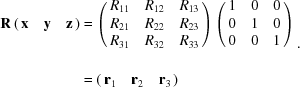Since the starting vectors xyz are orthogonal and of unit length, then the resultant vectors r1, r2, r3 must be also; these are the columns of the rotation matrix R.

#### 3.2. The transpose of a rotation matrix is its inverse

Premultiplying the rotation matrix R by its transpose RT (in which the columns of R become the rows of RT),Since r1, r2 and r3 are orthogonal unit vectors, then r1·r1 = 1, r1·r2 = 0 etc. (generally ri·rj = δij), thus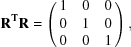hence RT = R−1, since this is the definition of an inverse matrix R−1.

#### 3.3. The rows of a rotation matrix are orthogonal unit vectors

This follows from §§3.1 and3.2, since the inverse (transposed) matrix must also be a rotation matrix, representing a rotation in exactly the opposite direction.

#### 3.4. The determinant of a rotation matrix = +1

The determinant of a matrix is the triple product of its column vectors, r1·(r2 × r3). This also corresponds to the volume of the parallelopiped with these vectors as edges. If the columns are orthogonal unit vectors, the determinant will be ±1. Considering the equation in §3.1, the volume of the parallelopiped defined by the unit matrix is +1, so for R to be a rotation matrix its determinant must also be +1. An orthogonal matrix with determinant −1 corresponds to an inversion: such a matrix may for example be obtained by interchanging any two columns or rows of a rotation matrix.

### 4. Rotations and angles

A rotational transformation is uniquely defined by a rotation matrix, but the natural expression of a rotation is as an angle; if we wish to enumerate all possible rotations for a systematic search, then angles are the usual way of doing this. However, a rotation may be expressed as angles in many different ways and this can be confusing. Within one program system it is not usually essential to understand the convention used, but it is essential when converting to another system. There are a number of programs available for converting conventions, including CONVROT (Urzhumtseva & Urzhumtsev, 1997) and ROTMAT from the CCP4 suite (Collaborative Computational Project, Number 4, 1994). Since the matrix itself is unique, conversions may be performed by generating the matrix from angles in one convention and decomposing the matrix into angles in the new convention.

In two dimensions, a single angle defines a rotation (Fig. 1a). We can write the transformation as a 2 × 2 matrix,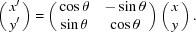Note that this already implies a convention: that θ is measured from x towards y. As an alternative to rotating the object, we can consider rotating the coordinate frame by an equal amount in the opposite direction, i.e by −θ (Fig. 1b). In using angles produced by computer programs, it is important to know which convention the author has used.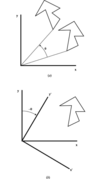Figure 1 (a) Rotation of an object in two dimensions by an angle θ. (b) Equivalent rotation of the axis system by −θ.

Extending this to three dimensions, we can write down rotation matrices for rotations around the principal axes xyz,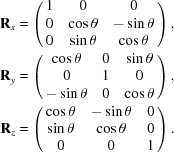### 5. Rotation angles in three dimensions

In three dimensions, we need three angles to describe a rotational transformation and to generate the corresponding rotation matrix, but this can be performed in many different ways. The different ways correspond to different starting points for measuring the angle, as in two dimensions, and also to alternative paths from the start point to the end point. Common angular descriptions fall into three classes.

 (i) Polar angles. Any rotation may be described as a single rotation around specified axis. One angle describes the rotation, the other two describe the direction of the axis. (ii) Eulerian angles. The rotation may be described as three successive rotations around principal axes: (a) the first and third rotations are around the same axes, e.g. rotate around z, then around the new y, then the new z or (b) the three rotations are around different axes, e.g. rotate around z, then the new y, then the new x.

#### 5.1. Polar angles

Polar angles are perhaps easier to visualize than Eulerian angles, but are algebraically less convenient for many purposes. The convention described is that used by the CCP4 programs and others: rotate by an angle κ around an axis whose direction is defined by angles ω (sometimes called ψ) and φ (Fig. 2). The direction of the axis may also be written as the components of a unit vector, the direction cosines lm and n,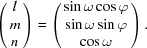The rotation matrix may be constructed by first rotating the rotation axis by −φ and −ω to put it along (say) the z axis, then rotating by κ around z, the finally rotating the axis back to its original position by ω and φ,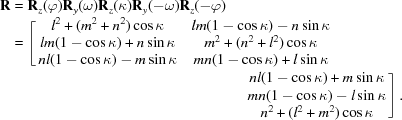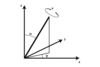Figure 2 Definition of polar angles, rotation by κ about an axis whose direction is defined by the angles ω and φ.
##### 5.1.1. Decomposing a matrix into polar angles

The rotation angle κ can be derived from the trace, i.e. the sum of the diagonal elements,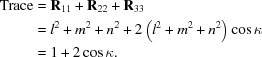In the general case, lm and n, and hence φ and ω, can be calculated from the off-diagonal elements: R32R23 = 2lsinκ; R13R31 = 2msinκ; R21 − R12 = 2nsinκ. Note that the full range of rotations can be generated with angles κ, ω, φ either in the range −π < κ < π, 0 < ω < π/2, 0 < φ < 2π or in the range 0 < κ < π, 0 < ω < π, 0 < φ < 2π, since the same matrix is generated by (−κ, πω, π + φ) as from (κωφ). This decomposition does not work if sinκ = 0 (i.e. κ = 0 or π). If κ = 0, there is no rotation and φ and ω can take any value. If κ = π (180°), then the matrix simplifies to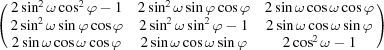and the off-diagonal elements are all zero for rotations of π around xy or z. Note that for a rotation of π or 180°, the matrix is symmetric: this must be so, since a rotation by +π is identical to a rotation by −π, so the rotation matrix is the same as its inverse, i.e. R = R−1 = RT.

All angular decompositions have special cases where some angles are indeterminate or where the simplest method of decomposition fails. This is typically when all off-diagonal terms are zero, i.e. either no rotation (the identity matrix) or a rotation of 180° around xy or z.

#### 5.2. Eulerian angles

Eulerian angles are algebraically simpler than polar angles, since we just rotate successively around three principal axes. For instance, the convention used in AMoRe (Navaza, 1994) and other CCP4 programs (Collaborative Computational Project, Number 4, 1994) is to rotate by γ around z, then by β around the new y, then by α around the new z again,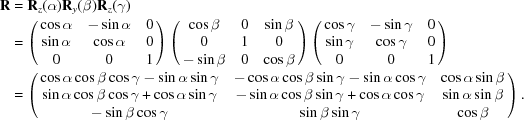Note that if β = 0 or π, the central matrix is diagonal, so rotations about α and γ have exactly the same effect (or exactly opposite effect if β = π). In these cases, only the combination α + γ or α − γ is determined.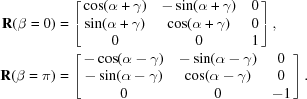### 6. Interaction with crystallographic symmetry

When superimposing a known model on an unknown structure in a crystal, equivalent solutions will be found for each molecule in the crystal, but it is not always obvious from the angles which alternate solutions are equivalent by crystallographic symmetry. Crystallographic symmetry is expressed as operations working on fractional cell coordinates, which are referred to crystal axes which are not necessarily orthogonal. For example, in Fig. 3, in space group P3, there are three solutions equivalent by the three crystallographic symmetry operations (xyz), (−y, xy, z) and (y − x, −x, z). To combine these operators with the rotation matrices defining the solution, which work on orthogonal coordinates, we need the conversion matrices from orthogonal coordinates xo to fractional coordinates xf and its inverse, such that xf = Fxo. In the most common convention (x, y, z along a, c* × a, c*),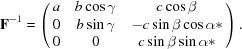Then, if the rotation from the model to molecule 1 is given by R, the three solutions are given by Rj = F−1SjFR, for j = 1, 3, where Sj is the matrix corresponding to the jth crystallographic symmetry operator, e.g.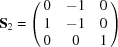in this case. Computer programs will usually identify solutions related by crystallographic symmetry or just present the user with a unique set.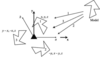Figure 3 An example of crystallographic symmetry: space group P3. The directions of the orthogonal axes are x along a, y along c* × a, z along c*. There are three possible solutions related by the threefold axis.

### 7. Non-crystallographic symmetry

Multiple solutions to a molecular-replacement search may also be produced by non-crystallographic symmetry. Unlike crystallographic symmetry, this is not generally known in advance, so no special relationship can be assumed. A common special case arises when a non-crystallographic rotation is parallel or nearly parallel to a crystallographic axis of the same order. In this case, the combination creates a non-crystallographic translation relating all atoms, which should be detectable in the native Patterson function. Either axis may contain a screw component. For example, in Fig. 4the combination of a crystallographic screw dyad and a non-crystallographic dyad creates a pure translation. The native Patterson function should always be examined for non-crystallographic translations.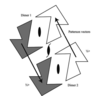Figure 4 A non-crystallographic dyad axis parallel to a crystallographic screw dyad gives rise to a non-crystallographic translation which will produce a peak in the native Patterson function. Molecules related by this translation are shaded in the same way.

### 8. Conclusions

Descriptions of rotations in terms of three angles can be confusing and in all conventions the angles have inconvenient properties of non-equivalence in the variables and singularities at certain points. Alternative descriptions exist in terms of variables other than angles, such as `rotation vectors' or quaternions; these may have advantages in some circumstances, but have been less commonly used than angle descriptions. Three alternative descriptions are discussed, for example, by Diamond (1993).

### ReferencesBIOLOGICALCRYSTALLOGRAPHY